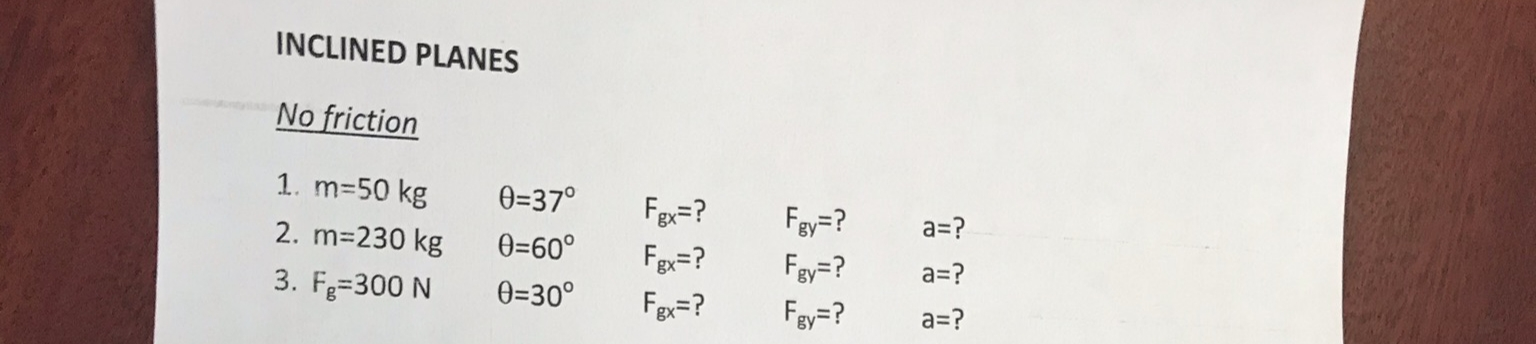# INCLINED PLANESNo friction1. m=50 kg0=37°Fgx=?Fgy=?Fgy=?a=?2. m=230 kg0=60°F=?a=?3. Fg=300 N0=30°Fgx=?Fgy=?a=?

Question
12 views

Inclined plane #3help_outlineImage TranscriptioncloseINCLINED PLANES No friction 1. m=50 kg 0=37° Fgx=? Fgy=? Fgy=? a=? 2. m=230 kg 0=60° F=? a=? 3. Fg=300 N 0=30° Fgx=? Fgy=? a=? fullscreen
check_circle

Step 1

1)

The free body diagram for all the three arrangements are illustrated below.

Step 2

Here, Fgx is the force acting on the mass m , parallel to the incline, Fgy is the force acting on the mass m perpendicular to the incline.

From the free body diagram illustrated above, it can be inferred that,

Step 3

Substitute 50 kg for m, 37˚ for θ , 9.8 m/s2 for g and solve for Fgx an...

### Want to see the full answer?

See Solution

#### Want to see this answer and more?

Solutions are written by subject experts who are available 24/7. Questions are typically answered within 1 hour.*

See Solution
*Response times may vary by subject and question.
Tagged in